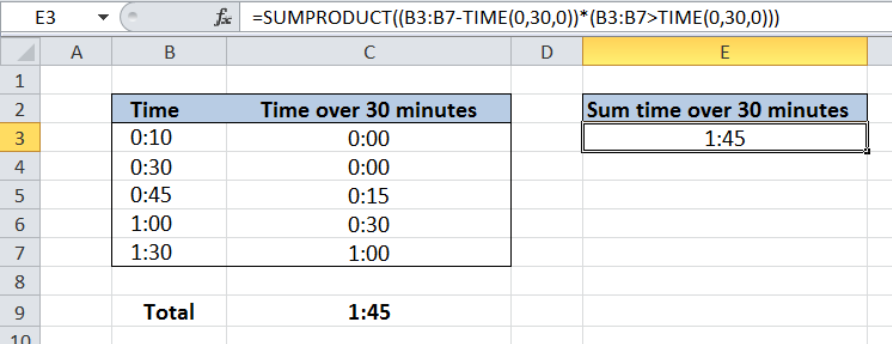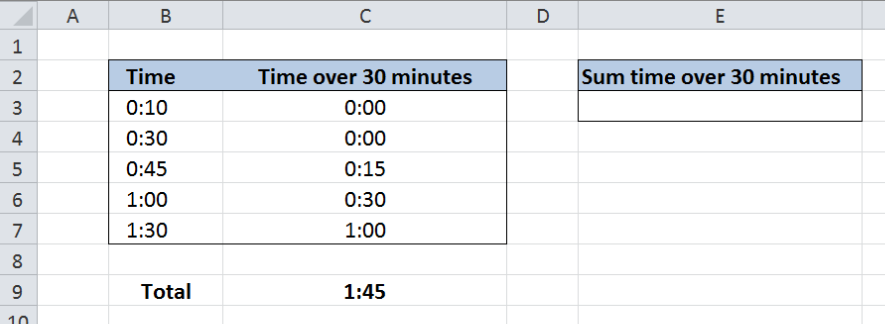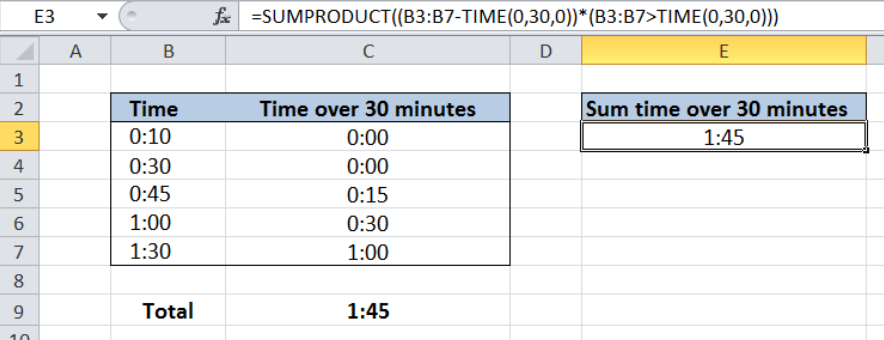Get instant live expert help with Excel or Google Sheets“My Excelchat expert helped me in less than 20 minutes, saving me what would have been 5 hours of work!”

#### Post your problem and you’ll get Expert help in seconds.

Your message must be at least 40 characters
Our professional Expert are available now. Your privacy is guaranteed.

# Sum time over 30 minutes

Performing operations involving time is tricky for most people but with Excel, it can be done rather easily with the use of key functions.  This step by step tutorial will assist all levels of Excel users in summing time over 30 minutes by using SUMPRODUCT and TIME functions.Figure 1. Final result: Sum time over 30 minutes

Final formula: `=SUMPRODUCT((B3:B7-TIME(0,30,0))*(B3:B7>TIME(0,30,0)))`

## Syntax of the SUMPRODUCT Function

SUMPRODUCT returns the sum of the products of two or more components in the given arrays

`=SUMPRODUCT(array1, [array2], [array3], ...)`

The parameters are:

• array – a range of values or cells whose values we want to multiply with other values and then add
• All array arguments must be in the same size
• Non-numeric values in an array are treated as zeros

## Syntax of TIME Function

TIME function is a built-in function in Excel that returns the decimal value of a particular time.  It is very useful in calculations involving time because it allows compartmentalization of time into hours, minutes and seconds.

`=Time(hour, minute, second)`

• When using this formula, we need to enter the time in this specific order : hours, minutes and then seconds

## Setting up the Data

Our table has two columns: Time (column B) and Time over 30 minutes (column C).  Column C represents the time in column B less 30 minutes.

Note that for cells B3 and B4, the value in column C is zero, since both “0:10” and “0:30” do not exceed 30 minutes.  We only want to sum the times exceeding 30 minutes. Column C is not needed in our calculation but it is only added for easy comparison of the result of our formula.

We want to sum the times in excess of 30 minutes for the values in column B.  The result will be recorded in column E.Figure 2. Sample data to sum time over 30 minutes

## Sum time over 30 minutes

In order to sum the times over 30 minutes, we use the SUMPRODUCT and TIME functions.  Let us follow these steps:

Step 1. Select cell E3

Step 2. Enter the formula: `=``SUMPRODUCT((B3:B7-TIME(0,30,0))*(B3:B7>TIME(0,30,0)))`

Step 3. Press Enter

The range of our data is B3:B7.  Our SUMPRODUCT formula has two components.  First, it subtracts 30 minutes from the time in column B by using the TIME function: (B3:B7TIME(0,30,0)).

Note that 30 minutes is represented by TIME(0,30,0).

The second component evaluates if the time in column B is greater than 30 minutes (B3:B7>TIME(0,30,0)) and returns an array of TRUE or FALSE for each item.  The resulting array is: `{FALSE;FALSE;``TRUE;TRUE;TRUE}`

Combining the first and second components result to this array: {0;0;0:15;0:30;1:00}. SUMPRODUCT then sums the resulting values and shows the result in cell E3, which is one hour and 45 minutes or “1:45”.Figure 3. Output: Using SUMPRODUCT and TIME to sum time over 30 minutes

Most of the time, the problem you will need to solve will be more complex than a simple application of a formula or function. If you want to save hours of research and frustration, try our live Excelchat service! Our Excel Experts are available 24/7 to answer any Excel question you may have. We guarantee a connection within 30 seconds and a customized solution within 20 minutes.

### Did this post not answer your question? Get a solution from connecting with the expert.Another blog reader asked this question today on Excelchat:
Solution examplesUse the Vlookup Function to complete the "employee" column of table 2. Use "job Id" from table 2 as your lookup_value(s) and table 1 as your reference.
Solved by C. H. in 16 minsIf a cell in another sheet is populated I need a vlookup done. If the cell is not populated I need the cell to return blank.
Solved by T. D. in 60 minsI am trying to make a chart that turns a week range red if nothing is entered in the range. If something is entered then I would like it to turn green. Please Help
Solved by E. U. in 43 minsI need a check box to show/hide an answer of an if function
Solved by Z. U. in 23 minsI need a formula to compare the data in two columns and then export the mismatched data in the 3rd column
Solved by S. Q. in 20 mins## Subscribe to Excelchat.coAnother blog reader asked this question today on Excelchat: